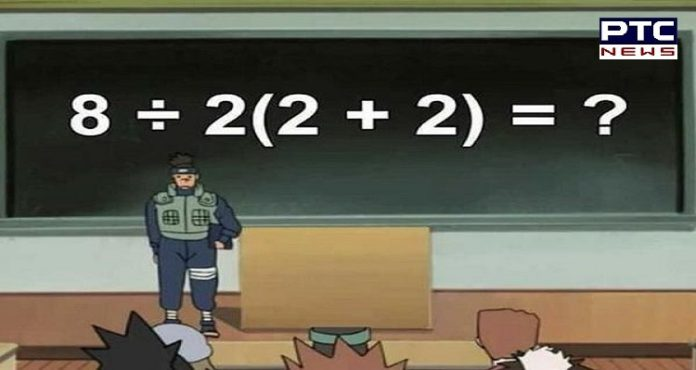# What does ‘8 ÷ 2(2 + 2)’ equal to? Can you solve this? Math equation goes viralWhat does '8 ÷ 2(2 + 2)' equal to? Can you solve this? Math equation goes viral

A Match equation is going viral again, where people despite getting a correct answer are scratching their heads. Well, this is because the equation has not a single answer, as various answers can be concluded using various formulas. The equation is 8 ÷ 2(2 + 2)=?.

Can you really solve this? Well, give it a try as there are many who have been trying to solve it, but many of them are facing difficulty. To be frank, the equation is easy, but Math has always been a rival and hence the answer needs a correct explanation with the answer. A twitter user posted this math equation and asked others to solve this.
A twitter user said, “Its 1. You have to do what’s in the parentheses first (PEMDAS)”

Well, one of the users accepted his fate in Mathematics and said, “i see so apparently division comes first……this is why i didn’t do well in math okay”

Also, he said, “math is dumb anyway follow me and watch naruto”. Likewise, another user said, “It’s 1. Blowing my mind how people are really debating this it’s fucking 3rd-grade math”.

Another user tried to crack it and said, “BODMAS rule is the key to solve arithmetical equations. Hence: the correct answer is 1.”

The actual answer will come out of the basic rule been taught in the school, i.e. BODMAS rule (Brackets, Orders, Division, Multiplication, Addition, Subtraction), which concludes the answer to be 16. However, if you use the PEMDAS rule (Parentheses, Exponents, Multiplication, Division, Addition, Subtraction), you will get the answer as 1.

-PTC News